Time Limit : sec, Memory Limit : KB

# Problem C: Pollock's conjecture

The nth triangular number is defined as the sum of the first n positive integers. The nth tetrahedral number is defined as the sum of the first n triangular numbers. It is easy to show that the nth tetrahedral number is equal to n(n+1)(n+2) ⁄ 6. For example, the 5th tetrahedral number is 1+(1+2)+(1+2+3)+(1+2+3+4)+(1+2+3+4+5) = 5×6×7 ⁄ 6 = 35.

The first 5 triangular numbers 1, 3, 6, 10, 15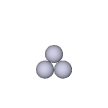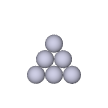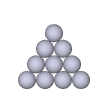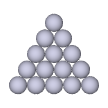The first 5 tetrahedral numbers 1, 4, 10, 20, 35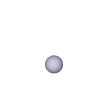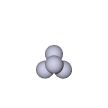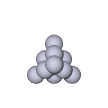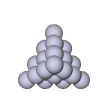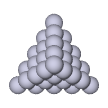In 1850, Sir Frederick Pollock, 1st Baronet, who was not a professional mathematician but a British lawyer and Tory (currently known as Conservative) politician, conjectured that every positive integer can be represented as the sum of at most five tetrahedral numbers. Here, a tetrahedral number may occur in the sum more than once and, in such a case, each occurrence is counted separately. The conjecture has been open for more than one and a half century.

Your mission is to write a program to verify Pollock's conjecture for individual integers. Your program should make a calculation of the least number of tetrahedral numbers to represent each input integer as their sum. In addition, for some unknown reason, your program should make a similar calculation with only odd tetrahedral numbers available.

For example, one can represent 40 as the sum of 2 tetrahedral numbers, 4×5×6 ⁄ 6 + 4×5×6 ⁄ 6, but 40 itself is not a tetrahedral number. One can represent 40 as the sum of 6 odd tetrahedral numbers, 5×6×7 ⁄ 6 + 1×2×3 ⁄ 6 + 1×2×3 ⁄ 6 + 1×2×3 ⁄ 6 + 1×2×3 ⁄ 6 + 1×2×3 ⁄ 6, but cannot represent as the sum of fewer odd tetrahedral numbers. Thus, your program should report 2 and 6 if 40 is given.

### Input

The input is a sequence of lines each of which contains a single positive integer less than 106. The end of the input is indicated by a line containing a single zero.

### Output

For each input positive integer, output a line containing two integers separated by a space. The first integer should be the least number of tetrahedral numbers to represent the input integer as their sum. The second integer should be the least number of odd tetrahedral numbers to represent the input integer as their sum. No extra characters should appear in the output.

### Sample Input

40
14
5
165
120
103
106
139
0


### Output for the Sample Input

2 6
2 14
2 5
1 1
1 18
5 35
4 4
3 37